# What are Logarithms, Bases and Exponents?

Eugene is a qualified control/instrumentation engineer Bsc (Eng) and has worked as a developer of electronics & software for SCADA systems.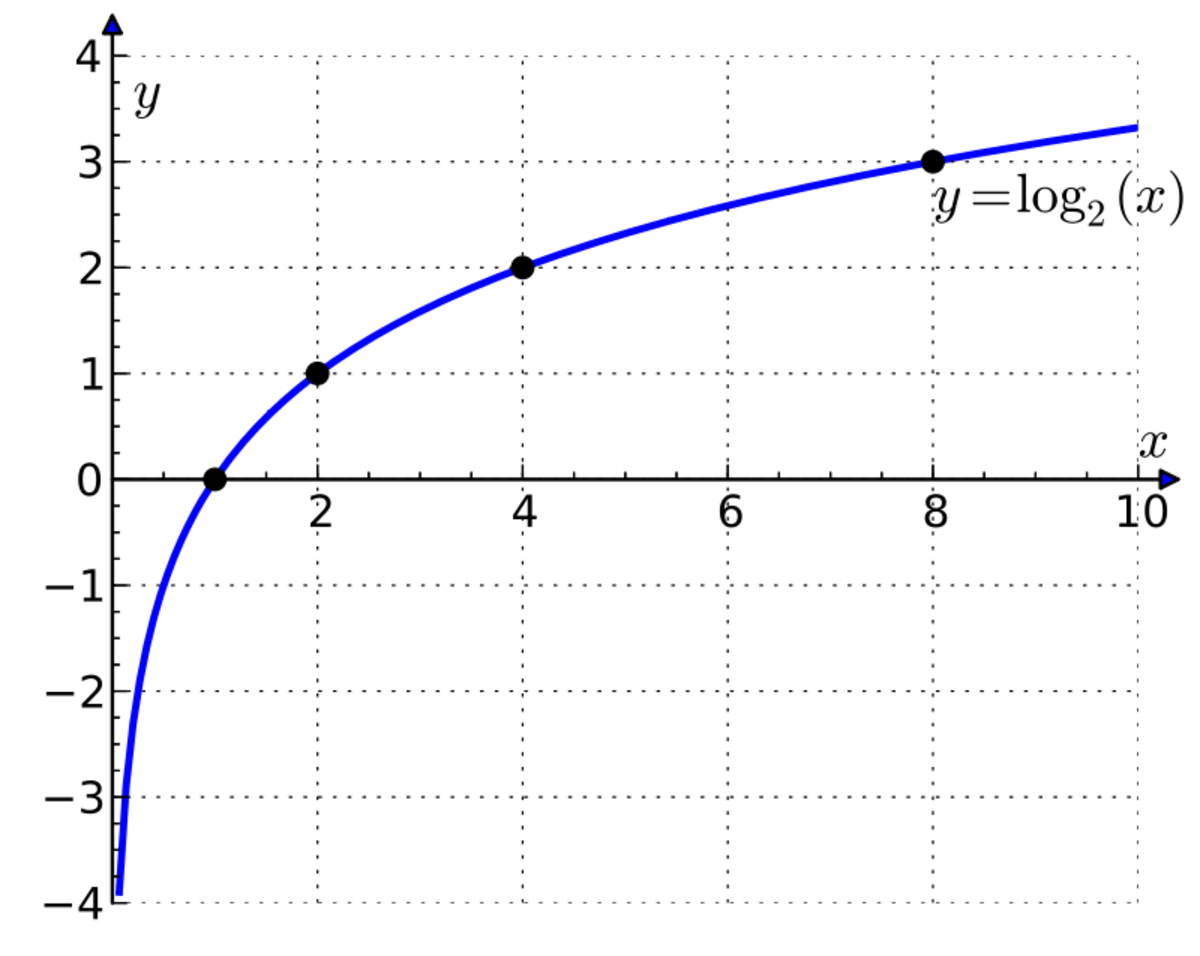A graph of a log function.Krishnavedala , CC BY-SA 3.0 via Wikimedia Commons

## An Introduction to Logarithms, Bases and Exponents

In this tutorial you'll learn about

• exponentiation
• bases
• logarithms to the base 10
• natural logarithms
• rules of exponents and logarithms
• working out logarithms on a calculator
• graphs of logarithmic functions
• the uses of logarithms

## What is Exponentiation?

Before we learn about logarithms, we need to understand the concept of exponentiation. Exponentiation is a math operation that raises a number to a power of another number to get a new number.

So 102 = 10 x 10 = 100

Similarly 43 = 4 x 4 x 4 = 64

and 25 = 2 x 2 x 2 x 2 x 2 = 32

We can also raise numbers with decimal parts (non-integers) to a power.

So 1.52 = 1.5 x 1.5 = 2.25

## What are Bases and Exponents?

In general, if b is an integer:

Then ab = a x a x a x a....a = c

a is called the base and b is called the exponent. As we'll find out later, b doesn't have to be an integer and can be a decimal.

## How to Simplify Expressions Involving Exponents

There are several laws of exponents (sometimes called "rules of exponents") we can use to simplify expressions that include numbers or variables raised to a power.

## Laws of Exponents

Scroll to Continue

## Examples Using the Laws of Exponents

50 = 1
270 = 1
10000 = 1

### Negative exponent

2-4 = 1/24 = 1/16

10-3 = 1/103 = 1/1000

### Product law

52 x 53 = 5 (2 + 3) = 55 = 3125

### Quotient law

34 / 32 = 3 (4 - 2) = 32 = 9

### Power of a power

(23)4 = 212 = 4096

### Power of a product

(2 x 3)2 = 62 = 36 = (22 x 32) = 4 x 9 = 36

## Exercise A: Laws of Exponents

Simplify the following:

1. yaybyc
2. papb/pxpy
3. papb/qxqy
4. ((ab)4)3 x ((ab)2)3
5. ( ((ab)4)3 x ((ab)4)3 )2 / a25

## Non-Integer Exponents

Exponents don't have to be integers, they can also be decimals.

For instance imagine if we have a number b, then the product of the square roots of b is b

So √b x √b = b

Now instead of writing √b we write it as b raised to a power x:

Then √b = bx and bx x bx = b

But using the product rule and the quotient of one rule we can write:

bx x bx = b2x = b = b1

So b2x = b1 Therefore 2x = 1 and x = 1/2

So √b = bx = b1/2

## What are Logarithms?

If we raise 10 to the power of 3, we get 1000.

103 = 10 x 10 x 10 = 1000

The logarithm function is the reverse of exponentiation and the logarithm of a number (or log for short) is the number a base must be raised to, to get that number.

So log10 1000 = 3 because 10 must be raised to the power of 3 to get 1000.

We indicate the base with the subscript 10 in log10 . Sometimes this is omitted.

### Some more examples:

102 = 100 and log10100 = 2

104 = 10,000 and log10 10,000 = 4

106 = 1000,000 and log10 1000,000 = 6

## Logarithms to Bases Other than 10

We can of course work out logs to other bases.

### Some examples:

23 = 8 and log2 8 = 3

34 = 81 and log3 81 = 4

## How to Work Out Logarithms Using a Calculator

You can use the log function on a calculator to work out the log of a number to the base 10.

1. Press "log".
2. Type the number.
3. You may have to press "=" depending on the model of the calculator.

To work out the log of a number to a base other than 10:

1. Press the "2nd function" or "shift" key
2. Press the "logy x" key
3. Type the base
4. Type the number
5. You may have to press "=" depending on the model of the calculator.

Not all calculators have a "logy x" key, so see "change of base" in properties of logarithms below.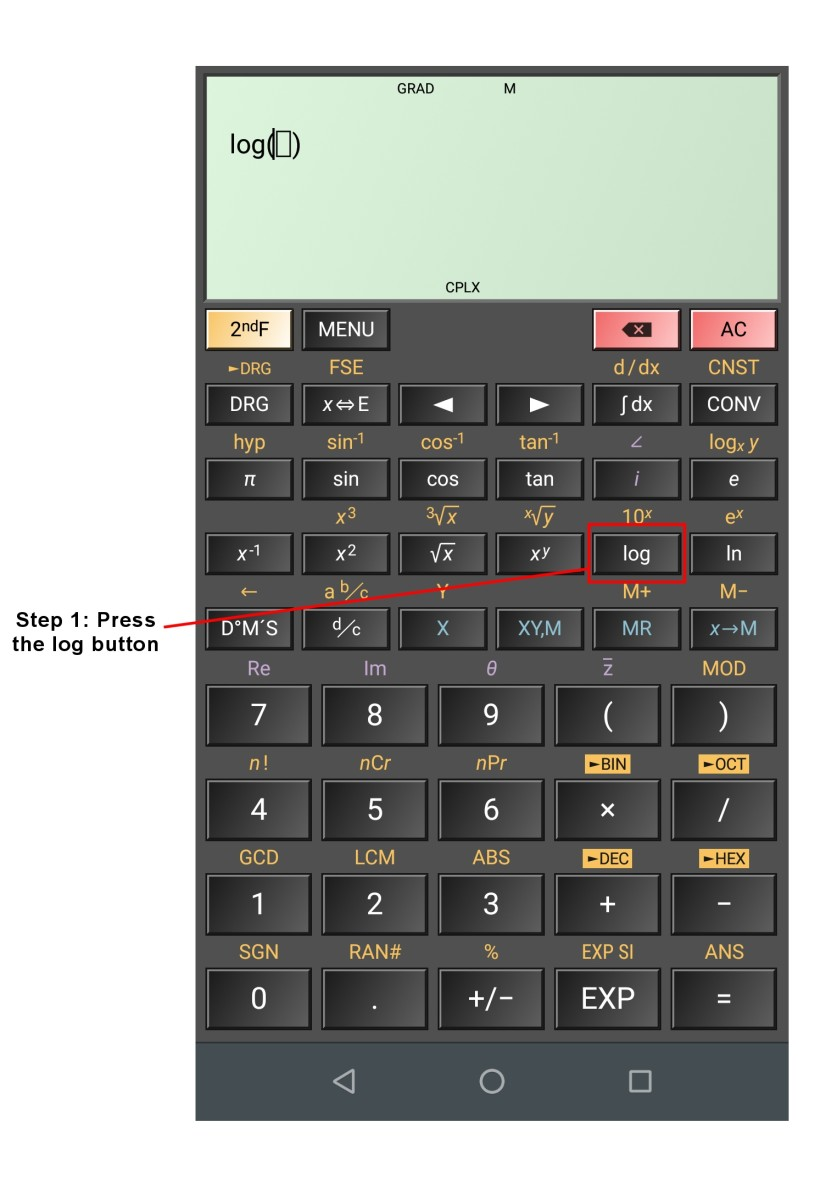Working out the log of a number to the base 10 using an Android calculator app.© Eugene Brennan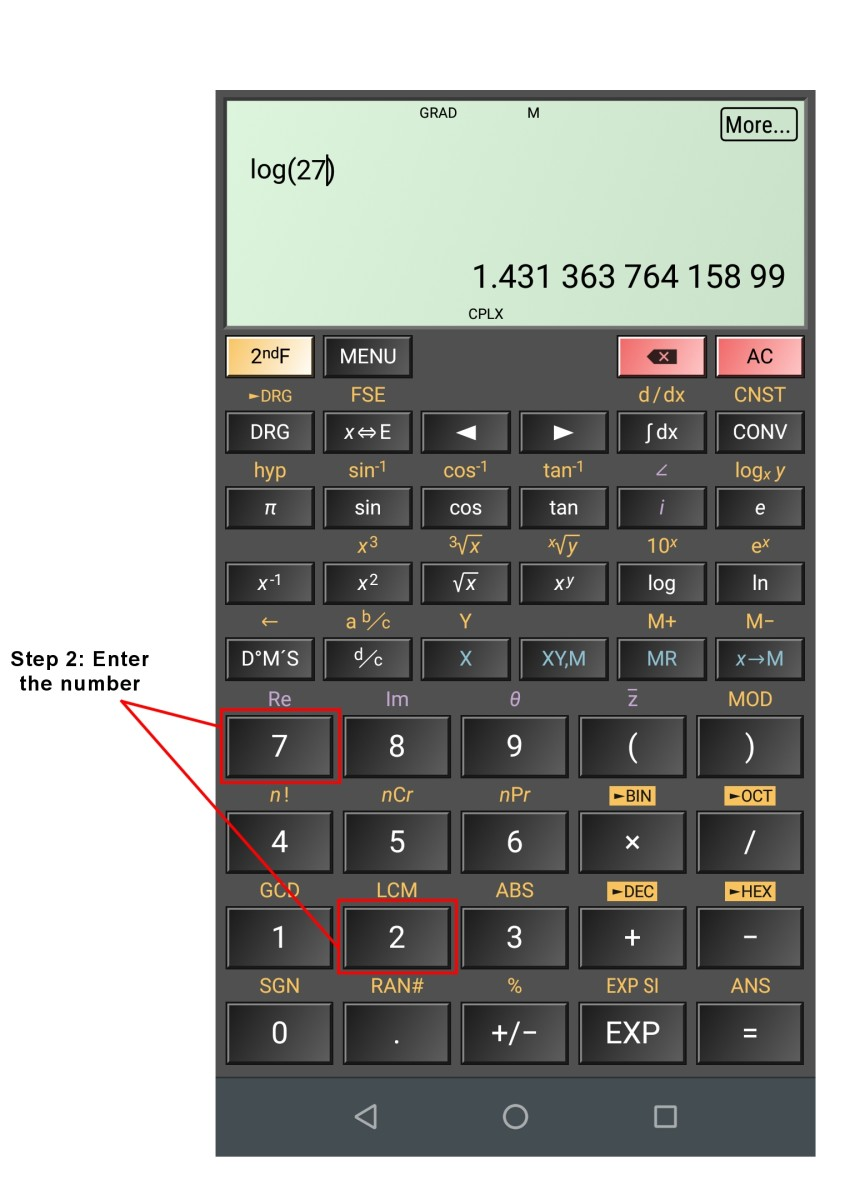Working out the log of a number to the base 10 using an Android calculator app. Some calculators require you to press "=" after you enter the number.© Eugene Brennan

## Exercise B: Calculate Values of Logs

Calculate the value of these logs:

1. log2256
2. log101000,000
3. log381
4. log1.5 3.375
5. ln 20.0855

## The Natural Logarithm

The mathematical constant e known as Euler's number is approximately equal to 2.71828

The value of the expression (1 + 1/n)n approaches e as n gets bigger and bigger.

The derivative of ex is itself. So d/dx(ex) = ex

The log of a number x to the base e is normally written as ln x or logex

## Graph of the Log Function

The graph below shows the function log (x) for the bases 10, 2 and e.

We notice several properties about the log function:

• Since x0 = 1 for all values of x, log (1) for all bases is 0.
• Log x increases at a decreasing rate as x increases.
• Log 0 is undefined. Log x tends to -∞ as x tends towards 0.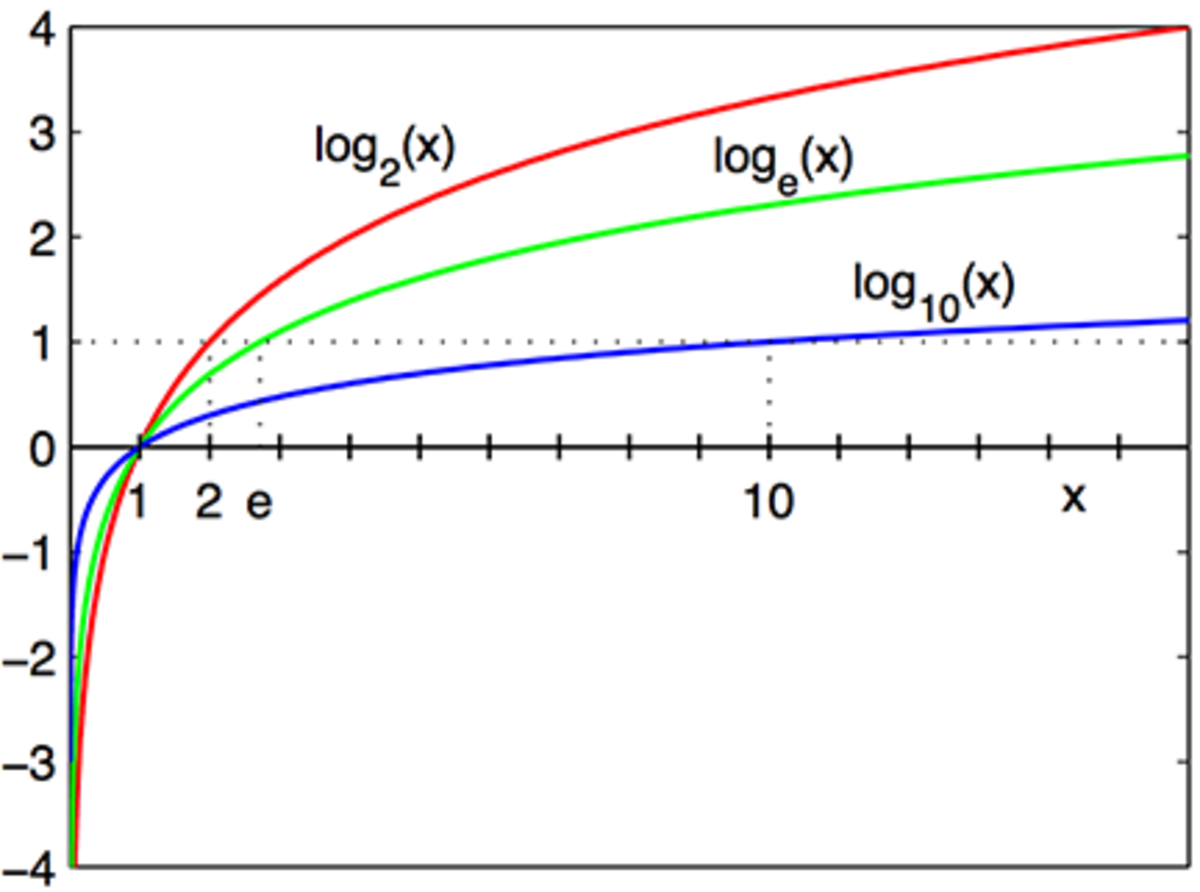Graph of the log x to various bases.Richard F. Lyon, CC by SA 3.0 via Wikimedia Commons

## Properties of Logarithms

These are sometimes called logarithmic identities or logarithmic laws.

• ### The product rule:

The log of a product equals the sum of the logs.

logc (AB) = logc A + logc B

• ### The quotient rule:

The log of a quotient (i.e. a ratio) is the difference between the log of the numerator and the log of the denominator.

logc (A/B) = logc A - logc B

• ### The power rule:

The log of a number raised to a power is the product of the power and the number.

logc (Ab) = blogcA

• ### Change of base:

logc A = logb A / logbc

This identity is useful if you need to work out a log to a base other than 10. Many calculators only have "log" and "ln" keys for log to the base 10 and natural log to the base e respectively.

Example:

What is log2256 ?

log2256 = log10256 / log102 = 8

## Exercise C: Using Rules of Logs to Simplify Expressions

Simplify the following:

1. log10 35x
2. log10 5/x
3. log10x5
4. log10 10x3
5. log2 8x4
6. log3 27(x2/y4)
7. log5 (1000) in terms of the base 10, rounded to two decimal places

## What are Logarithms Used For?

• Representing numbers with a large dynamic range
• Compressing scales on graphs
• Multiplying and dividing decimals
• Simplifying functions to work out derivatives

## Representing Numbers With a Large Dynamic Range

In science, measurements can have a large dynamic range. This means that there can be a huge variation between the smallest and largest value of a parameter.

### Sound pressure levels

An example of a parameter with a large dynamic range is sound.

Typically sound pressure level (SPL) measurements are expressed in decibels.

Sound pressure level = 20log10 ( p / p0)

where p is the pressure and po is a reference pressure level (20 μPa, the faintest sound the human ear can hear)

By using logs, we can represent levels from 20 μPa = 20 x 10-5 Pa up to the sound level of a rifle gunshot (7265 Pa) or higher on a more usable scale of 0dB to 171dB.

So if p is 20 x 10-5, the faintest sound we can hear

Then SPL = 20log10 ( p / p0)

= 20log10 ( 20 x 10-5 / 20 x 10-5)

= 20log10 (1) = 20 x 0 = 0dB

If sound is 10 times louder , i.e. 20 x 10-4

Then SPL = 20log10 ( p / p0)

= 20log10 ( 20 x 10-4 / 20 x 10-5)

= 20log10 (10) = 20 x 1 = 20dB

Now increase the sound level by another factor of 10, i.e. make it 100 times louder than the faintest sound we can hear.

So p = 20 x 10-3

SPL = 20log10 ( p / p0)

= 20log10 ( 20 x 10-3 / 20 x 10-5)

= 20log10 (100) = 20 x 2 = 40dB

So each 20DB increase in SPL represents a tenfold increase in level of sound pressure.

### Richter magnitude scale

The magnitude of an earthquake on the Richter scale is determined by using a seismograph to measure the amplitude of ground movement waves. The log of the ratio of this amplitude to a reference level gives the strength of the earthquake on the scale.

The original scale is log10 ( A / A0 ) where A is the amplitude and A0 is the reference level. Similar to sound pressure measurements on a log scale, every time the value on the scale increases by 1, this represents a tenfold increase in strength of the earthquake. So an earthquake of strength 6 on the Richter scale is ten times stronger than a level 5 earthquake and 100 times stronger than a level 4 quake.

## Logarithmic Scales on Graphs

Values with a large dynamic range are often represented on graphs with nonlinear, logarithmic scales. The x-axis or y-axis or both can be logarithmic, depending on the nature of data represented. Each division on the scale normally represents a tenfold increase in value. Typical data displayed on a graph with a logarithmic scale is:

• Sound pressure level (SPL)
• Sound frequency
• Earthquake magnitudes (Richter scale)
• pH (acidity of a solution)
• Light intensity
• Tripping current for circuit breakers and fuses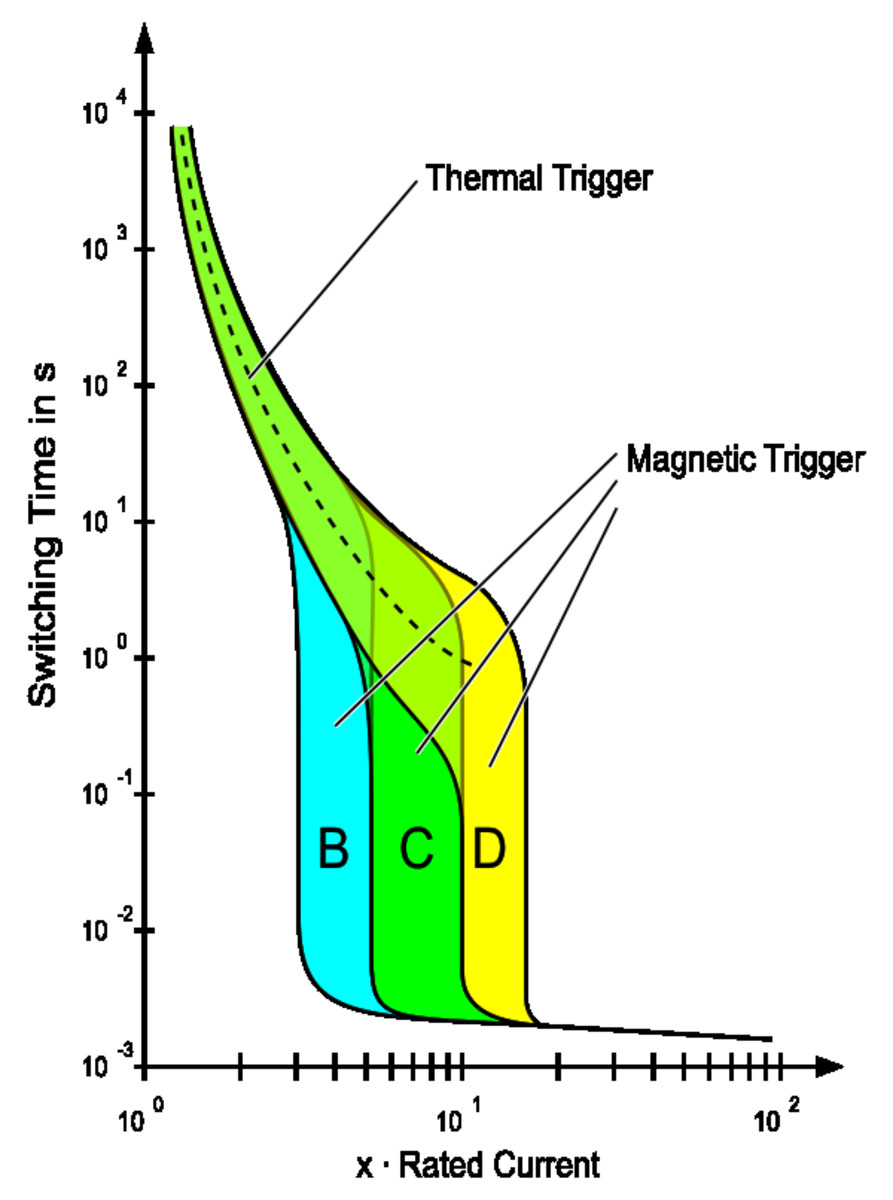Trip current for an MCB protective device. (These are used to prevent cable overload and overheating when excess current flows). The current scale and time scale are logarithmic.Public domain image via Wikimedia Commons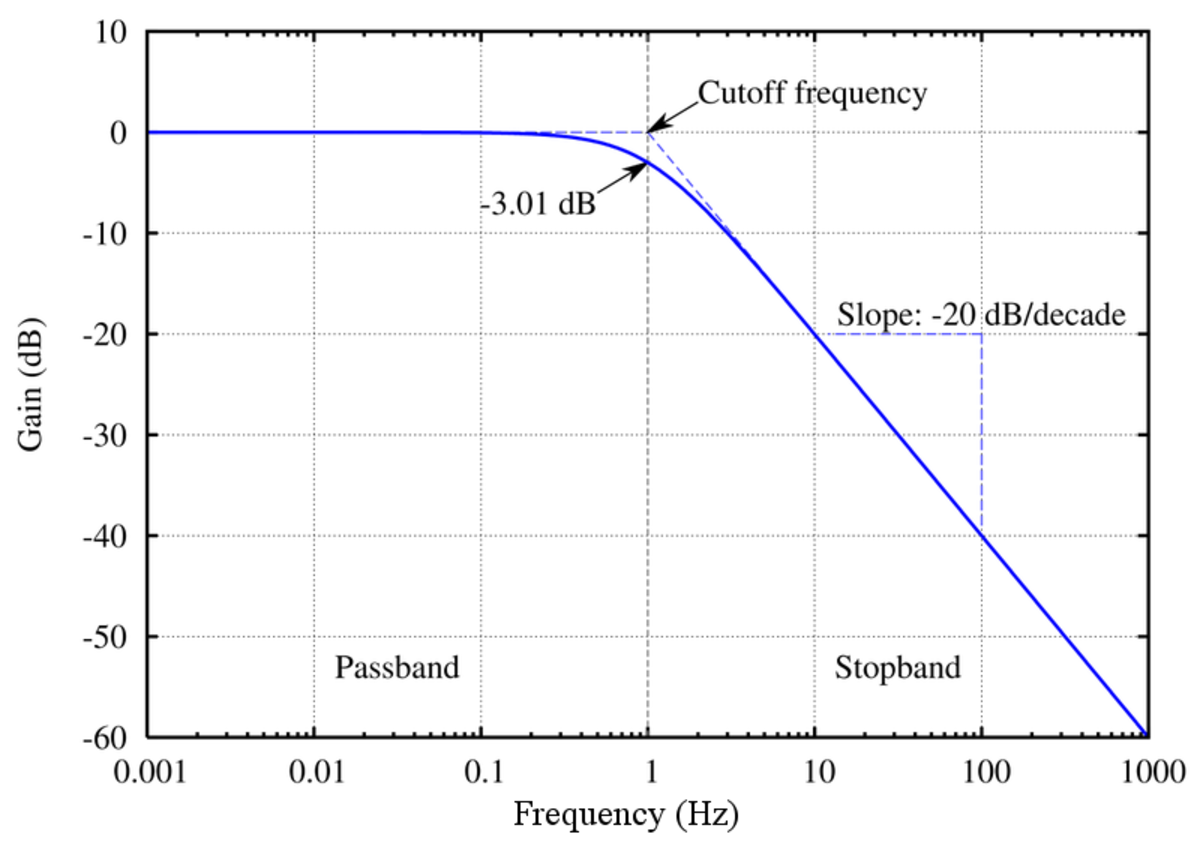Frequency response of a low pass filter, a device that only allows low frequencies through below a cut-off frequency (e.g. audio in a sound system). The frequency scale on the x axis and gain scale on the y axis are logarithmic.Original unedited file Omegatron, CC by SA 3.0

Exercise A

1. y(a + b +c)
2. p(a + b -x - y)
3. p(a +b/q
4. (ab)18
5. a23b48

Exercise B

1. 8
2. 6
3. 4
4. 3
5. 3

Exercise C

1. log10 35 + log10 x
2. log10 5 - log10x
3. 5log10x
4. 1 + 3log10x
5. 3 + 4log2x
6. 3 + 2log3x - 4log3y
7. log10 1000 / log10 5 = 4.29 approx

This content is accurate and true to the best of the author’s knowledge and is not meant to substitute for formal and individualized advice from a qualified professional.